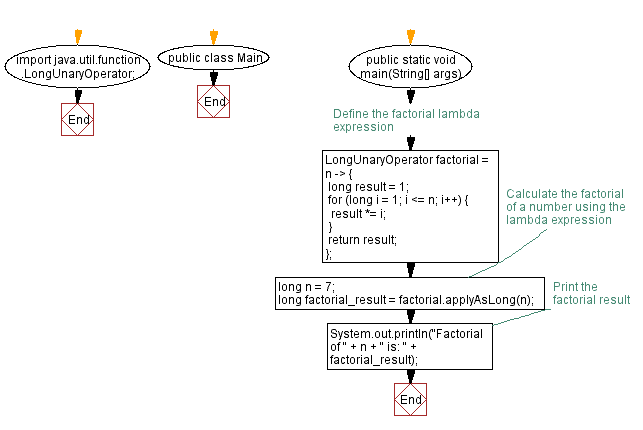# Calculate factorial using Lambda expression in Java

## Java Lambda Program: Exercise-8 with Solution

Write a lambda expression to implement a lambda expression to calculate the factorial of a given number.

Sample Solution:

Java Code:

``````import java.util.function.LongUnaryOperator;

public class Main {
public static void main(String[] args) {
// Define the factorial lambda expression
LongUnaryOperator factorial = n -> {
long result = 1;
for (long i = 1; i <= n; i++) {
result *= i;
}
return result;
};

// Calculate the factorial of a number using the lambda expression
long n = 7;
long factorial_result = factorial.applyAsLong(n);

// Print the factorial result
System.out.println("Factorial of " + n + " is: " + factorial_result);
}
}
``````

Sample Output:

```Factorial of 7 is: 5040
```

Explanation:

In the above exercise, we define a lambda expression using the LongUnaryOperator functional interface. This interface represents an operation on a single long operand and produces a long result.

In the main method, we create an instance of the LongUnaryOperator using the lambda expression. Then, we specify the number for which the factorial should be calculated.

In order to calculate the factorial, we use the applyAsLong method of the lambda expression and pass the number as an argument. In the factorialResult variable, we store the result.

Lastly, we print the factorial result using System.out.println ().

Flowchart:Live Demo:

Java Code Editor:

Improve this sample solution and post your code through Disqus

Java Lambda Exercises Previous: Remove duplicates from list of integers using Lambda expression in Java.
Java Lambda Exercises Next: Check prime number using Lambda expression in Java.

What is the difficulty level of this exercise?

Test your Programming skills with w3resource's quiz.

﻿

## Java: Tips of the Day

Hashset vs Treeset:

HashSet is much faster than TreeSet (constant-time versus log-time for most operations like add, remove and contains) but offers no ordering guarantees like TreeSet.

HashSet

• the class offers constant time performance for the basic operations (add, remove, contains and size).
• it does not guarantee that the order of elements will remain constant over time
• iteration performance depends on the initial capacity and the load factor of the HashSet.
• It's quite safe to accept default load factor but you may want to specify an initial capacity that's about twice the size to which you expect the set to grow.

TreeSet

• guarantees log(n) time cost for the basic operations (add, remove and contains)
• guarantees that elements of set will be sorted (ascending, natural, or the one specified by you via its constructor) (implements SortedSet)
• doesn't offer any tuning parameters for iteration performance
• offers a few handy methods to deal with the ordered set like first(), last(), headSet(), and tailSet() etc

Important points:

• Both guarantee duplicate-free collection of elements
• It is generally faster to add elements to the HashSet and then convert the collection to a TreeSet for a duplicate-free sorted traversal.
• None of these implementations are synchronized. That is if multiple threads access a set concurrently, and at least one of the threads modifies the set, it must be synchronized externally.
• LinkedHashSet is in some sense intermediate between HashSet and TreeSet. Implemented as a hash table with a linked list running through it, however,it provides insertion-ordered iteration which is not same as sorted traversal guaranteed by TreeSet.

So a choice of usage depends entirely on your needs but I feel that even if you need an ordered collection then you should still prefer HashSet to create the Set and then convert it into TreeSet.

• e.g. SortedSet<String> s = new TreeSet<String>(hashSet);

Ref: https://bit.ly/3d3waGh

We are closing our Disqus commenting system for some maintenanace issues. You may write to us at reach[at]yahoo[dot]com or visit us at Facebook